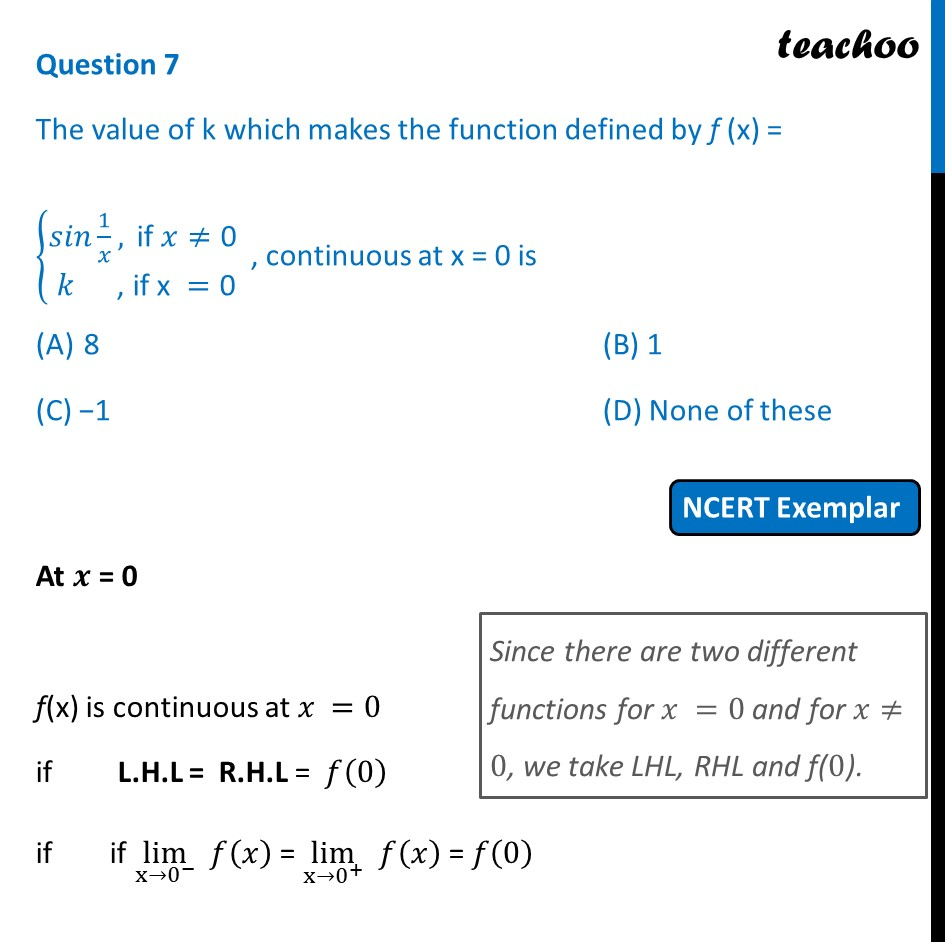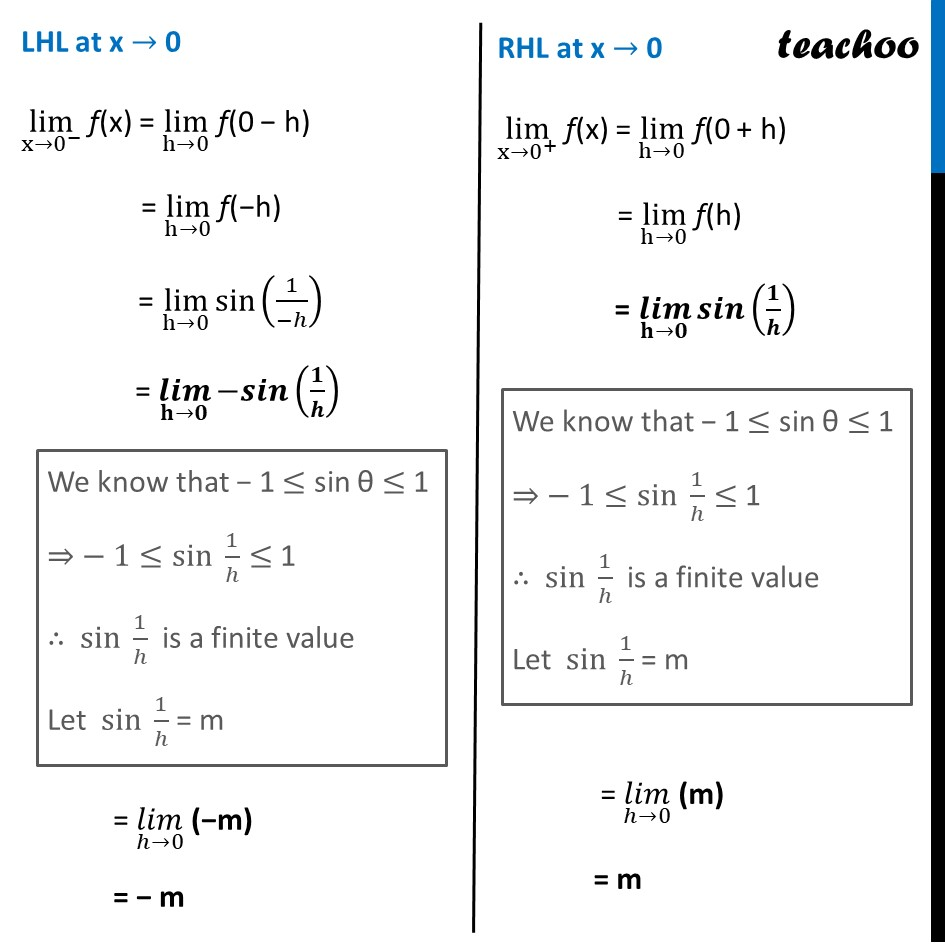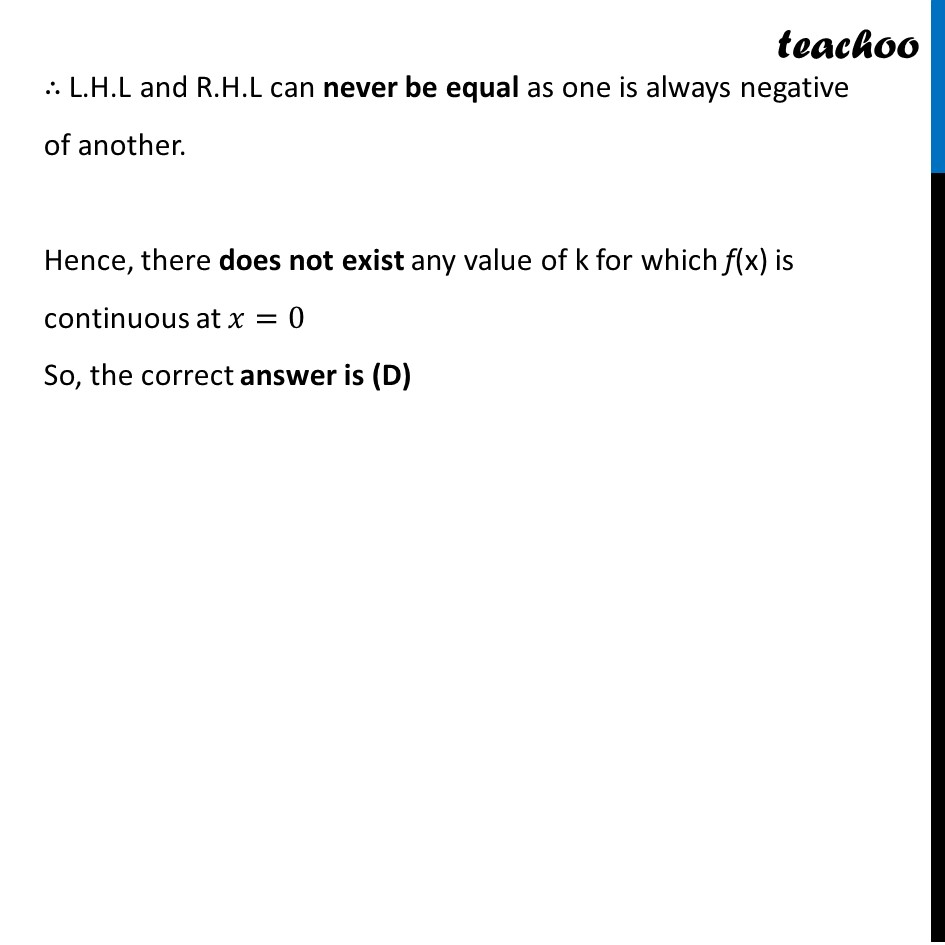NCERT Exemplar - MCQs

Chapter 5 Class 12 Continuity and Differentiability
Serial order wise

## (D) None of these

This question is similar to Ex 5.1, 24 - Chapter 5 Class 12 - Continuity and DifferentiabilityLearn in your speed, with individual attention - Teachoo Maths 1-on-1 Class

### Transcript

Question 7 The value of k which makes the function defined by f (x) = {■8(𝑠𝑖𝑛 1/𝑥," if " 𝑥≠"0 " @𝑘 ", if x " ="0" )┤ , continuous at x = 0 is 8 (B) 1 (C) −1 (D) None of these At 𝒙 = 0 f(x) is continuous at 𝑥 =0 if L.H.L = R.H.L = 𝑓(0) if if lim┬(x→0^− ) 𝑓(𝑥) = lim┬(x→0^+ ) 𝑓(𝑥) = 𝑓(0) LHL at x → 0 lim┬(x→0^− ) f(x) = lim┬(h→0) f(0 − h) = lim┬(h→0) f(−h) = lim┬(h→0) sin⁡(1/(−ℎ)) = (𝒍𝒊𝒎)┬(𝐡→𝟎) 〖−𝒔𝒊𝒏〗⁡(𝟏/𝒉) = (𝑙𝑖𝑚)┬(ℎ→0) (−m) = − m RHL at x → 0 lim┬(x→0^+ ) f(x) = lim┬(h→0) f(0 + h) = lim┬(h→0) f(h) = (𝒍𝒊𝒎)┬(𝐡→𝟎) 𝒔𝒊𝒏⁡(𝟏/𝒉) = (𝑙𝑖𝑚)┬(ℎ→0) (m) = m ∴ L.H.L and R.H.L can never be equal as one is always negative of another. Hence, there does not exist any value of k for which f(x) is continuous at 𝑥=0 So, the correct answer is (D)